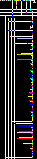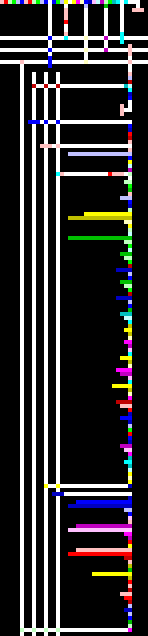# Fibonacci numbers in Piet

Example for versions npiet 1.2

This example was generated automatically. The original program translated into the image follows; it uses iterative calculation of Fibonacci numbers.

``````main()
{
f1 = 0;
f2 = 1;
for ( i = 1; i <= 16; i++ )
{
__outn(f2);
__out(44);
__out(32);
f2 = f1 + f2;
f1 = f2 - f1;
}
__out(46);
__out(46);
__out(46);
__out(10);
}
``````Fibonacci numbers in Piet (autogenerated)Fibonacci numbers in Piet (autogenerated, 4x scale)